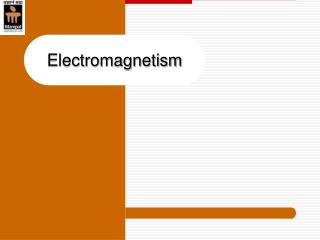DownloadDownload PresentationElectromagnetism

# Electromagnetism

Download Presentation## Electromagnetism

- - - - - - - - - - - - - - - - - - - - - - - - - - - E N D - - - - - - - - - - - - - - - - - - - - - - - - - - -
##### Presentation Transcript

1. Electromagnetism

2. + - Magnetic field around a current carrying conductor Grip rule : If the conductor is gripped with right hand, with the thumb extended in the direction of current, the fingers wrapped around the conductor indicate the direction of field. Magneticflux Dept of E&E,MIT Manipal

3. Field Magnetic field produced by a solenoid Grip rule: If the coil (solenoid) is gripped with the right hand, with the fingers pointing in the direction of current, then the thumb out- stretched parallel to the axis of the coil points in the direction of the magnetic fieldinside the solenoid Dept of E&E,MIT Manipal

4. Force acting on a Conductor • A current carrying conductor, placed in a magnetic field, produces a force. • This force can be increased, • When the current in the conductor is increased • When length of conductor is increased • When the magnetic field is made stronger • Force on the conductor= f lux density  length of conductor  current or f = B I l Newton Note: considering single conductor Dept of E&E,MIT Manipal

5. thumb (Mechanical force) (First finger) Field (Second finger) Current Direction of Force: Fleming’s left hand rule Mechanical force exerted on the conductor always acts in a direction perpendicular to the plane of the conductor and the magnetic field This is the principle of electric motor Dept of E&E,MIT Manipal

6. Electromagnetic Induction An EMF is induced in a conductor if the following conditions are satisfied: • Magnetic field • Conductor in the vicinity of magnetic field • Magnetic field linking with conductor changes OR Relative motionbetween the Conductor & Field Faraday’s laws of Electromagnetic Induction • When a conductor cuts or it is cut by magnetic flux, an emf is induced in the conductor. • The magnitude of the induced emf is proportional to the rate at which the conductor cuts or is cut by the magnetic flux Dept of E&E,MIT Manipal

7. Electromagnetic Induction • Direction (Polarity) of induced emf: • Lenz’s law: • An electro magnetically induced emf always acts in such a direction to set up a current opposing the motion or change of flux responsible for inducing the emf. Dept of E&E,MIT Manipal

8. Motion Field (thumb) (First finger) Emf (second finger) Fleming's right hand rule Right hand rule: If the first finger of the right hand is pointed in the direction of the magnetic flux, and if the thumb is pointed in the direction of motion of the conductor relative to the magnetic field, then the second finger, held at right angles to both the thumb and the first finger represents the direction of emf. Dept of E&E,MIT Manipal

9. Induced emf • Statically induced emf: - The voltage induced in the conductor due to change in the magnetic field • Conductor is stationary • Magnetic Field is changing in a stationary Magnetic System; • Ex: Transformer • Dynamically induced emf: - The voltage induced in the conductor due to relative motion of conductor and magnetic field • Conductor is moving/stationary • Magnetic Field is stationary/moving Dept of E&E,MIT Manipal